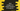# Java example program to create one ArrayList of ArrayList# How to create one ArrayList of ArrayList in Java :

In this quick Java programming tutorial, I will show you how to create one ArrayList of ArrayList, i.e. an ArrayList with ArrayList elements.  The program will take all inputs from the user. It will take the ArrayList inputs and then print out the result.

## Java program to create ArrayList of ArrayList :

``````import java.util.ArrayList;
import java.util.Scanner;

public class Example {
public static void main(String[] args) {
//1
ArrayList<ArrayList> myList = new ArrayList<>();

//2
int arrayListCount, itemCount;
Scanner scanner = new Scanner(System.in);

//3
System.out.println("Enter total number of ArrayList to add : ");
arrayListCount = scanner.nextInt();

//4
System.out.println("Enter total values for each ArrayList : ");
itemCount = scanner.nextInt();

//5
for (int i = 0; i < arrayListCount; i++) {
//6
System.out.println("Enter all values for ArrayList " + (i + 1) + " : ");
ArrayList list = new ArrayList<>();
//7
for (int j = 0; j < itemCount; j++) {
//8
System.out.println("Enter value " + (j + 1) + " : ");
list.add(scanner.next());
}
//9
myList.add(list);
}

//10
System.out.println(myList);
}
}``````

### Explanation of the above Java ArrayList of ArrayList program :

The commented numbers in the above program denote the step numbers below :

1. Create one ArrayList of ArrayList myList. This will hold ArrayList elements and each ArrayList can hold string elements.
2. Create two integer variables: arrayListCount to hold the total count of ArrayList and itemCount to hold the total count of strings for each ArrayList. We can also have ArrayList with different size. Create one Scanner variable scanner to read the user inputs.
3. Ask the user to enter the total number of ArrayList to add. Read the value and store it in arrayListCount.
4. Ask the user to enter the total elements for each ArrayList. Read it and store it in itemCount.
5. Run one for loop to get inputs of all ArrayList.
6. Ask the user to enter all values for the current ArrayList. Create one ArrayList variable list.
7. Run one for-loop for itemCount time to get all values for the current ArrayList.
8. Ask the user to enter the current value for the ArrayList. Read it and add it to the ArrayList.
9. Add the ArrayList to the ArrayList of ArrayList.
10. Finally, print out the ArrayList of ArrayList.

### Sample Output :

``````Enter total number of ArrayList to add :
3
Enter total item numbers for each ArrayList :
2
Enter values for ArrayList 1 :
Enter item 1 :
a
Enter item 2 :
b
Enter values for ArrayList 2 :
Enter item 1 :
c
Enter item 2 :
d
Enter values for ArrayList 3 :
Enter item 1 :
e
Enter item 2 :
f
[[a, b], [c, d], [e, f]]``````

You can also download the above example from here.